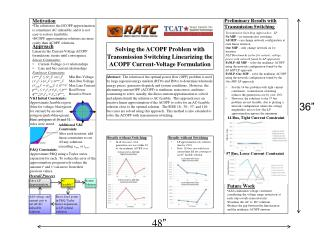DownloadDownload Presentation48 ”

# 48 ”

Télécharger la présentation## 48 ”

- - - - - - - - - - - - - - - - - - - - - - - - - - - E N D - - - - - - - - - - - - - - - - - - - - - - - - - - -
##### Presentation Transcript

1. Preliminary Results with Transmission Switching • Motivation • The solution to the DCOPF approximation is sometimes AC infeasible, and it is not easy to restore feasibility. • DCOPF approximation solutions are more costly than ACOPF solutions. • Transmission Switching Approaches: LP • No MIP – no transmission switching • All MIP– can change network configuration at each linear iteration • One MIP – only change network on 1st iteration • NLP Benchmarks (solve for current, voltage, power with network found by LP approach) • FxNLP-All MIP– solve the nonlinear ACOPF using the network configuration found by the All MIP LP approach. • FxNLP-One MIP – solve the nonlinear ACOPF using the network configuration found by the One MIP LP approach. • For the 14 bus problem with tight current constraints, transmission switching reduces the generation cost by over 10%. • However, the solutions to the 57 bus problem are not feasible, due to picking a network configuration where the voltage magnitudes are too low (the LP approximation ignores the minimum voltage requirements). • Approach • Linearize the Current-Voltage ACOPF formulation; iterate until convergence. • Linear Constraints: • Current-Voltage (i-v) relationships • Line and bus current relationships • Nonlinear Constraints • (vminn)2 ≤ (vrn)2 +(vjn)2 Min Bus Voltage • (vrn)2 +(vjn)2 ≤ (vmaxn)2Max Bus Voltage • (irnmk)2 + (ijnmk)2 ≤ (imax)2MaxLineCurrent • pminn≤ vrnirn+vjnijn≤ pmaxReal Power qminn≤vjnirn-vrnijn≤ qmaxn Reactive Power Solving the ACOPF Problem with Transmission Switching Linearizing the ACOPF Current-Voltage Formulation Abstract: The solution of the optimal power flow (OPF) problem is used by large regional energy markets (RTOs and ISOs) to determine wholesale energy prices, generator dispatch, and system conditions.  However, the alternating current OPF (ACOPF) is nonlinear, nonconvex, and time-consuming to solve; usually, the direct-current approximation is solved and adjusted until the solution is AC feasible.  This approach uses an iterative linear approximation of the ACOPF to solve for an AC-feasible solution close to the optimal solution.  The IEEE 18-, 30-, 57- and 118- bus cases are solved using this approach.  This method is also extended to solve the ACOPF with transmission switching. V&I Initial Constraints: Approximate feasible region (blue for voltage, blue+green for current) by an outer polygon (pink+blue+green). Here, polygons of 16 and 32 sides were tested. 36” 14 Bus, Tight Current Constraint Additional V&I Constraints: After each iteration, add linear constraints to cut off any solutions exceeding vmaxor imax. Objective Value Results without Switching Results without Switching • In all test cases, total generation cost was within 4% of the nonlinear ACOPF cost. • LP approximation cuts solution time by >50% • Note: 118 bus case with loose current limit did not converge to an AC feasible solution. P&Q Constraints: Approximate P&Q using a Taylor series expansion for each. To reduce the error of the approximation, progressively reduce the amount vr and vi can move from their previous values. 57 Bus, Loose Current Constraint Overall Process Solve LP Approximation AC Feasible? Yes Return Solution • Future Work • Add a minimum voltage constraint , considering the voltage range restriction at each step (avoids nonconvexity) • Examine the AC vs. DC solutions • Reduce the gap between the linearization and the nonlinear ACOPF answers No Add voltage and current cuts to cut off AC infeasible solutions Reset fixed points in P&Q Taylor series expansions as LP optimal solutions 48”